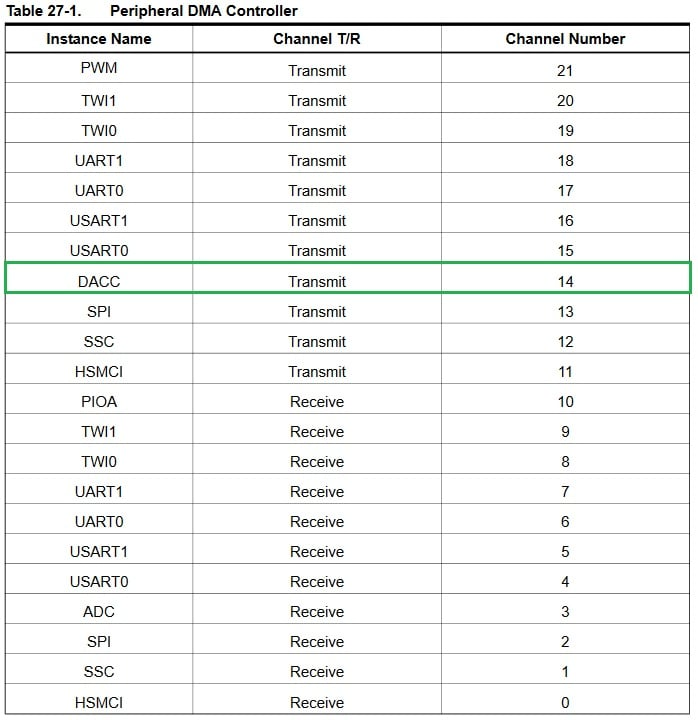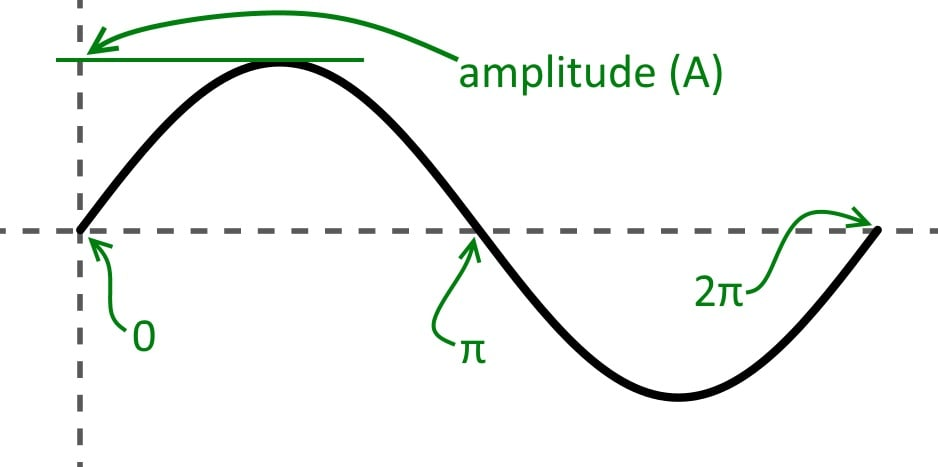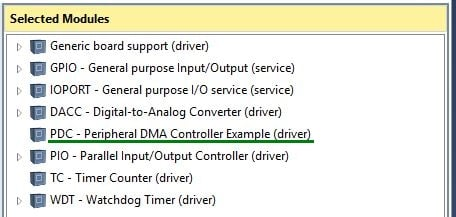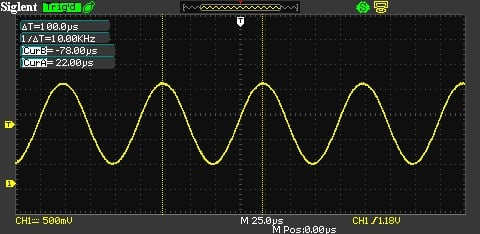Project

# Digital-to-Analog Conversion with the SAM4S Peripheral DMA Controller

May 09, 2016 by Robert Keim

## Part Three in this three-article series shows you how to generate values for a discrete sinusoid and continuously convert this data into an analog signal without overburdening the CPU.

Part Three in this three-article series shows you how to generate values for a discrete sinusoid and continuously convert this data into an analog signal without overburdening the CPU.

### Don’t Stress Out Your Processor

In the previous article, we completed the somewhat arduous process of configuring the digital-to-analog converter controller (DACC), and then we used an infinite while loop to continuously generate a triangle wave. This is by no means an insignificant accomplishment, but at the same time, it would be far from ideal to burden the CPU with all the instructions needed to continue this DAC activity.

Let’s say we use a DAC sample rate of 1 MHz; if the microcontroller has other important tasks to perform, it would be seriously inconvenient for the processor to be interrupted one million times per second and forced to execute the various instructions needed to access an array, increment a counter, load data into the DACC’s conversion-data register, or perform any other tasks that might be needed in a particular application.

Of course, chip designers have long recognized this problem—why clog up a sophisticated microprocessor with something as simple as moving data from one memory location to another? Why not incorporate another “processor” that knows little more than how to move data around, and let this subprocessor lighten the CPU’s load? Well, it turns out that this is indeed a very good idea, and these data-moving subprocessors—referred to as direct memory access (DMA) controllers—have been incorporated into numerous microcontrollers and digital signal processors.

In this stage of the project, we will add DMA functionality to our digital-to-analog conversion by making use of the SAM4S peripheral DMA controller (PDC). With the help of this module, we can move sine-wave values from RAM to the DACC’s conversion-data register with minimal CPU intervention. The following table, from page 491 of the SAM4S datasheet (PDF), lists the peripherals that are supported by the PDC:The “channel numbers” on the right indicate the priorities that the PDC uses when handling multiple transfer requests, with channel 0 being the highest priority. We don’t have to worry about any priority business in this project because we are using only one PDC peripheral. Note also that some channels support transmit and receive, meaning that you can use the PDC to, for example, move data to the UART for transmission and store data received by the UART.

### First, Some Trigonometry

Before we use the PDC to move sinusoid data to the DACC, we need sinusoid data. So let’s take a look at how to generate discrete-time sine-wave values. First we need to include a header file that will provide the mathematical functionality we need. We will use the “arm_math.h” file, which gives us the sin() function and defines PI as 3.14159265358979. Now let’s remind ourselves about some fundamental characteristics of a sine wave:When we use the sin() function, values corresponding to one full cycle require arguments ranging from 0 to 2π. The sin() function returns zero when the argument is 0, π, or 2π; it returns 1 (the maximum value) when the argument is exactly halfway between 0 and π (i.e., π/2), and it returns –1 (the minimum value) when the argument is exactly halfway between π and 2π (i.e., 3π/2). To create a sinusoid with maximum and minimum values different from 1 and –1, we multiply the sin() return values by a constant referred to as the amplitude. We can also give the sinusoid an offset—e.g., raise it above the x-axis so that no portion of the wave extends below zero—by adding a constant to all the data points that result from multiplying the return values by the amplitude.

So if we want to generate one sine-wave cycle, the first sin() argument should be 0 and the last should be 2π. But what do we do with all the arguments in between? Well, at this point we need to decide how many data points, aka samples, we want per sine-wave cycle. Then we divide 2π by the number of samples per cycle to determine how much the argument increases from one data point to the next.

The following code should help you to understand the entire discrete-sine generation process:


//variables for the discrete-time sine wave
uint16_t SineWave_12bit[SAMPLES_PER_CYCLE];
float DiscreteSineArgument, DiscreteSineArgumentStep;

DiscreteSineArgument = 0;
DiscreteSineArgumentStep = (2*PI)/SAMPLES_PER_CYCLE;

for (n = 0; n < SAMPLES_PER_CYCLE; n++)
{
SineWave_12bit[n] = (uint16_t)((SINE_AMPLITUDE * sin(DiscreteSineArgument)) + SINE_MIDRANGE);
DiscreteSineArgument = DiscreteSineArgument + DiscreteSineArgumentStep;
}




I like a nice smooth sine wave, so in this project we will use 100 samples per cycle. SINE_AMPLITUDE is set to 2047.5, i.e., half of the DAC’s 12-bit range. This means that the maximum value will be 2047.5 counts above the midrange value and the minimum value will be 2047.5 counts below the midrange value, and thus the sine wave will cover the DAC’s entire 4095-count output range.

SINE_MIDRANGE is also set to 2047.5 because we want the minimum value to be at 0 counts. (Usually 0 counts would correspond to an analog output voltage of 0 V, but in the case of the SAM4S DAC, 0 counts corresponds to one-sixth of the analog reference voltage. This issue is briefly discussed in the “Results and Conclusion” section of the previous article.)

### Back to the PDC

Here are the steps involved in firing up a PDC channel:

1. Add the PDC ASF module (I don’t know why it’s called “Peripheral DMA Controller Example”):1. Define a structure of type pdc_packet_t, used for PDC channel configuration, and a pointer variable of type PDC, used to point to the base address of the peripheral’s PDC register.
2. Load the relevant address into the base-address pointer using the function corresponding to the peripheral you are using; for this project, the function is dacc_get_pdc_base().
3. Use the pdc_packet_t structure to store the starting address and length for the DMA transfer. The length refers to bytes if you are moving 8-bit data, half-words if you are moving 16-bit data, and words if you are moving 32-bit data.
4. Configure the DMA channel for transmit or receive using pdc_tx_init() or pdc_rx_init() in conjunction with the pdc_packet_t structure.
5. Initiate the PDC transfer using pdc_enable_transfer().

The PDC automatically moves data at the proper time because its operation is governed by transmit and/or receive status signals controlled by the peripheral. For example, with the DACC, the PDC transfers new data only when the DACC’s transmit-ready signal indicates that the DAC hardware is ready to perform a new conversion.

The above process can be translated into code as follows:


//PDC variables
pdc_packet_t DACC_PDC_Config;
Pdc *PDC_ptr_to_DACC;

PDC_ptr_to_DACC = dacc_get_pdc_base(DACC);

DACC_PDC_Config.ul_size = SAMPLES_PER_CYCLE;
pdc_tx_init(PDC_ptr_to_DACC, &DACC_PDC_Config, &DACC_PDC_Config);

pdc_enable_transfer(PDC_ptr_to_DACC, PERIPH_PTCR_TXTEN);



### We Still Need the CPU

When the PDC has finished moving all the data from the buffer to the DACC, an interrupt is used to provoke CPU intervention; the processor needs to know that the PDC transfer is complete so that it can reconfigure the PDC channel and thereby ensure that sine-wave data keeps moving to the DACC. So, the last step involved in implementing the PDC is enabling the proper interrupt and setting up an interrupt service routine (ISR) that will execute when the PDC has completed the transfer.

Inside the ISR, we simply re-configure the PDC channel with the same buffer and transfer length. Thanks to the DACC’s integrated FIFO (discussed under “The Basics” in the previous article), the processor should have no difficulty in completing this re-configuration before the DACC runs out of conversion data.

Here is the interrupt-related code:


int main (void)
{
.  .  .

//enable the DACC's "end of transmit buffer" interrupt
dacc_enable_interrupt(DACC, DACC_IMR_ENDTX);

//enable DACC interrupts in the Nested Vectored Interrupt Controller
NVIC_EnableIRQ(DACC_IRQn);

.  .  .
}

void DACC_Handler(void)
{
//confirm that the "end of transmit buffer interrupt" fired
if (dacc_get_interrupt_status(DACC) & DACC_ISR_ENDTX)
{
//re-configure the PDC so that sine-wave generation continues
pdc_tx_init(PDC_ptr_to_DACC, &DACC_PDC_Config, &DACC_PDC_Config);
}
}



You might be wondering how Atmel Studio knows that the DACC_Handler() function is the DACC ISR—there’s nothing that explicitly identifies it as such. Well, it’s actually pleasantly simple: The function name “DACC_Handler” is the official identifier for the DACC’s ISR, so all you have to do is name the function DACC_Handler() and it will automatically be used as the ISR for any DACC interrupts. This same scheme applies to other peripherals—ADC_Handler(), UART0_Handler(), etc.

### One Buffer or Two?

When you configure a PDC channel using pdc_tx_init() or pdc_rx_init(), you have to specify the starting address and transfer length for the “current” transfer. However, you have the option of specifying a starting address and transfer length for the “next” transfer. In the above code, I configured the channel for both “current” and “next” transfers. To configure the channel for only one transfer, you use NULL for the third argument, as follows:

pdc_tx_init(PDC_ptr_to_DACC, &DACC_PDC_Config, NULL);


The advantage of using both transfers is that CPU intervention is reduced; the CPU re-configures the PDC channel after it moves two buffers instead of one. I suppose the two-transfer configuration would also be handy if you wanted to move data to or from one buffer then to or from a different buffer: You could set up both buffers with one call to pdc_rx_init() or pdc_tx_init(), thereby maintaining the proper sequence without the CPU being required to determine which buffer was just moved.

### Results

DMA_DAC_SineWave_Part3.zip

Here is a scope capture showing the sine wave generated by the DAC:Note that the frequency is 10 kHz. This is what we expect: The sample period is 1/(1 MHz) = 1 µs, and we have 100 samples per cycle. Thus, the sine-wave period is 100 µs, and 1/(100 µs) = 10 kHz. We can generalize this as follows:

$f_{sine}=\frac{sample\ rate}{samples\ per\ cycle}$

If you want a higher sine-wave frequency, you can either reduce the samples per cycle (resulting in a lower-quality waveform) or increase the sample rate (the SAM4S DAC’s maximum settling time is 0.5 µs, so you probably shouldn’t push the sample rate beyond 2 MHz).

### Conclusion

In this project series we took a detailed look at the timer/counter module, the digital-to-analog converter controller, the peripheral DMA controller, and some code for generating a discrete-time sine wave. This functionality could prove useful for a variety of applications. One such application—namely, generating baseband waveforms for a software-defined radio—will be the subject of a future article.